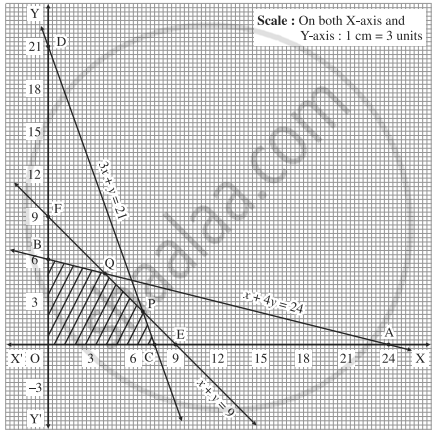Advertisement Remove all ads

# Find the feasible solution of the following inequation: x + 4y ≤ 24, 3x + y ≤ 21, x + y ≤ 9, x ≥ 0, y ≥ 0. - Mathematics and Statistics

Graph
Sum

Find the feasible solution of the following inequation:

x + 4y ≤ 24, 3x + y ≤ 21, x + y ≤ 9,  x ≥ 0, y ≥ 0.

Advertisement Remove all ads

#### Solution

First we draw the lines AB, CD and EF whose equations are x + 4y = 24, 3x + y = 21 and x + y = 9 respectively.

 Line Equation Points on the X-axis Points on the Y-axis Sign Region AB x + 4y = 24 A (24,0) B (0,6) ≤ origin side of line AB CB 3x + y = 21 C (7,0) D(0,21) ≤ origin side of line CD EF x + y = 9 E(9,0) F(0,9) ≤ origin side of line EFThe feasible solution is OCPQBO which is shaded in the graph.

Concept: Linear Programming Problem (L.P.P.)
Is there an error in this question or solution?
Advertisement Remove all ads

#### APPEARS IN

Advertisement Remove all ads
Advertisement Remove all ads
Share
Notifications

View all notifications

Forgot password?